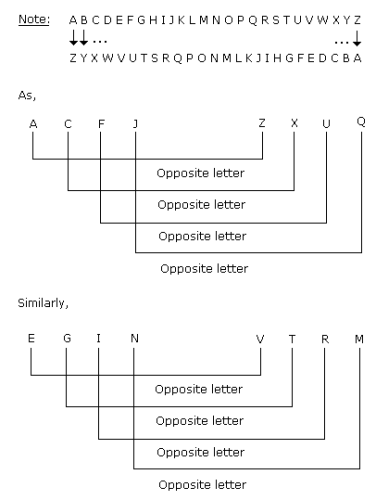Courses

# Test: Analogy- 2

## 20 Questions MCQ Test Logical Reasoning (LR) and Data Interpretation (DI) | Test: Analogy- 2

Description
This mock test of Test: Analogy- 2 for UPSC helps you for every UPSC entrance exam. This contains 20 Multiple Choice Questions for UPSC Test: Analogy- 2 (mcq) to study with solutions a complete question bank. The solved questions answers in this Test: Analogy- 2 quiz give you a good mix of easy questions and tough questions. UPSC students definitely take this Test: Analogy- 2 exercise for a better result in the exam. You can find other Test: Analogy- 2 extra questions, long questions & short questions for UPSC on EduRev as well by searching above.
QUESTION: 1

### Directions to Solve In each of the following questions find out the alternative which will replace the question mark. Question - BEGK : ADFJ :: PSVY : ?

Solution: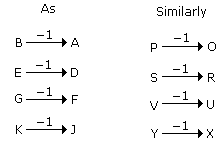QUESTION: 2

### Directions to Solve In each of the following questions find out the alternative which will replace the question mark. Question - AZBY : CXDW :: EVFU : ?

Solution: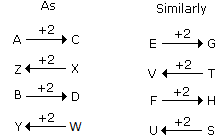QUESTION: 3

### Directions to Solve In each of the following questions find out the alternative which will replace the question mark. Question - ACEG : IKMO :: QSUW : ?

Solution: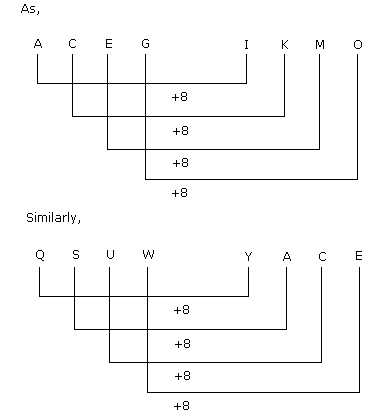QUESTION: 4

Directions to Solve

In each of the following questions find out the alternative which will replace the question mark.

Question -

BCFG : HILM :: NORQ : ?

Solution: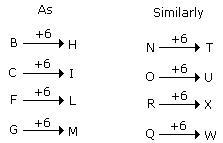QUESTION: 5

Directions to Solve

In each of the following questions find out the alternative which will replace the question mark.

Question -

ZRYQ : KCJB :: PWOV : ?

Solution: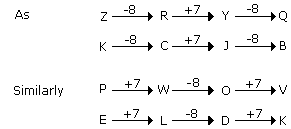QUESTION: 6

Directions to Solve

In each of the following questions find out the alternative which will replace the question mark.

Question -

KeaC : CaeK :: XgmF : ?

Solution:

First and fouth letters are interchanged while second and third letters are interchanged.

QUESTION: 7

Directions to Solve

In each of the following questions find out the alternative which will replace the question mark.

Question -

Corden : zrogbq :: ? : pxivro

Solution: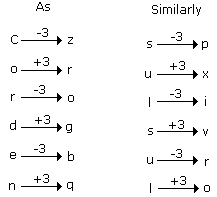QUESTION: 8

Directions to Solve

In each of the following questions find out the alternative which will replace the question mark.

Question -

LKJ : pon :: ? : hgf

Solution: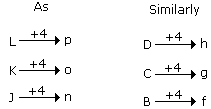QUESTION: 9

Directions to Solve

In each of the following questions find out the alternative which will replace the question mark.

Question -

Computer : fqprxvht :: Language : ?

Solution: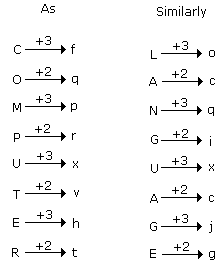QUESTION: 10

Directions to Solve

In each of the following questions find out the alternative which will replace the question mark.

Question -

AEFJ : KOPT :: ? : QUVZ

Solution: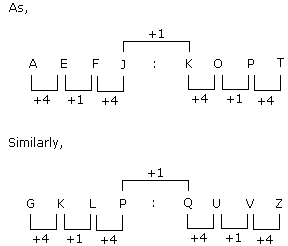QUESTION: 11

Directions to Solve

In each of the following questions find out the alternative which will replace the question mark.

Question -

PASS : QBTT :: FAIL : ?

Solution: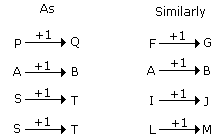QUESTION: 12

Directions to Solve

In each of the following questions find out the alternative which will replace the question mark.

Question -

BLOCKED : YOLXPVW :: ? : OZFMXS

Solution: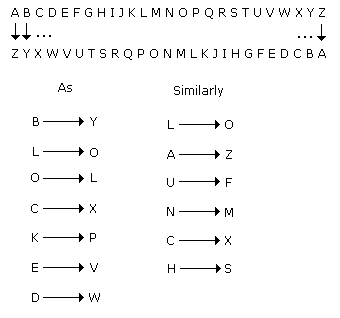QUESTION: 13

Directions to Solve

In each of the following questions find out the alternative which will replace the question mark.

Question -

CEDH : HDEC :: ? : PNRV

Solution:

CEDH -- Reverse it ---> HDEC.

VRNP -- Reverse it ---> PNRV.

Therefore VRNP is the answer.

QUESTION: 14

Directions to Solve

In each of the following questions find out the alternative which will replace the question mark.

Question -

DFHJ : LNPR :: TVXZ : ?

Solution: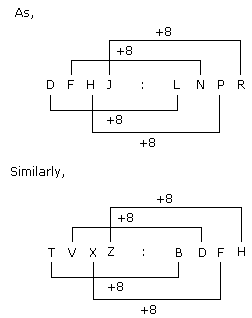QUESTION: 15

Directions to Solve

In each of the following questions find out the alternative which will replace the question mark.

Question -

ACEG : ? :: BDFH : KMOQ

Solution: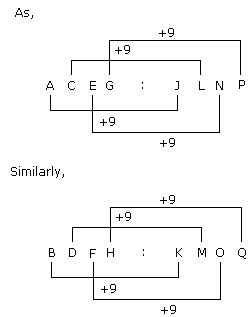QUESTION: 16

Directions to Solve

In each of the following questions find out the alternative which will replace the question mark.

Question -

REASON : SFBTPO :: THINK : ?

Solution: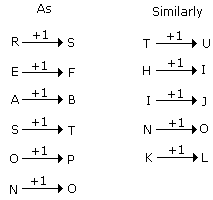QUESTION: 17

Directions to Solve

In each of the following questions find out the alternative which will replace the question mark.

Question -

PSQR : CFED :: JMKL : ?

Solution: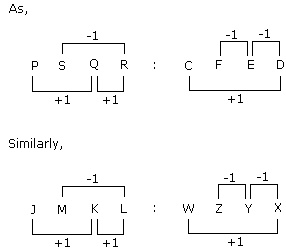QUESTION: 18

Directions to Solve

In each of the following questions find out the alternative which will replace the question mark.

Question -

FIELD : GJFME :: SICKLE : ?

Solution: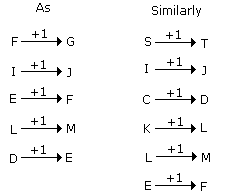QUESTION: 19

Directions to Solve

In each of the following questions find out the alternative which will replace the question mark.

Question -

? : QEHMDF :: WIDELY : HVCDXK

Solution: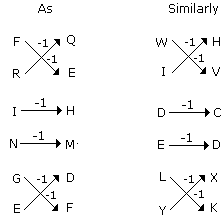QUESTION: 20

Directions to Solve

In each of the following questions find out the alternative which will replace the question mark.

Question -

ACFJ : ZXUQ :: EGIN : ?

Solution: